# Hillshade, colors and marginal plots with tidyterra (I)

How to overlay SpatRasters

This is the first post of a series of two, showing how to overlay a SpatRaster on top of a Hillshade background. Next post would show how to add marginal plots including information of the values of the raster by longitude and latitude. See the second post here.

Using shadow effects on relief mappings is a very common technique, that allows to produce informative yet beautiful maps. If you are interested on this topic and you work with R, you would have probably seen this map:The production of this map by Timo Grossenbacher has been a reference for years. However, last developments on the R package ecosystem (`terra`, `sf` and support of both classes on `ggplot2`, development of `ggnewscale`, etc.) can make even easier the task of producing such type of maps.

In fact, Dominic Royé recently wrote a very detailed post on creating shadow effects on map reliefs. On this first post of the series I would replicate that technique with a slight variation (e.g. not making use of `ggnewscale`) and I would discuss a bit on the potential choice of a color palette for this kind of maps.

## Libraries

I would use the following libraries:

``````
## Libraries

library(terra)
library(tidyterra)
library(ggplot2)
library(dplyr)
library(scales)

# Get the data
library(geodata)
``````

## Get the data

First step is to get the altitude data. I use here the package `geodata` for simplicity, but you can use as well `elevatr` that is much more complete. However `elevatr` produces the result as `RasterLayers`, so you would need to convert the object to `SpatRaster` with `terra::rast()`.

``````
# Cache map data
mydir <- "~/R/mapslib/misc"

r_init <- elevation_30s("ROU", path = mydir)

r_init
#> class       : SpatRaster
#> dimensions  : 588, 1176, 1  (nrow, ncol, nlyr)
#> resolution  : 0.008333333, 0.008333333  (x, y)
#> extent      : 20.1, 29.9, 43.5, 48.4  (xmin, xmax, ymin, ymax)
#> coord. ref. : lon/lat WGS 84 (EPSG:4326)
#> source      : ROU_elv_msk.tif
#> name        : ROU_elv_msk
#> min value   :          -4
#> max value   :        2481

# For better handling we set here the names
names(r_init) <- "alt"

# We don't want values lower than 0
r <- r_init %>%
mutate(alt = pmax(0, alt))

r
#> class       : SpatRaster
#> dimensions  : 588, 1176, 1  (nrow, ncol, nlyr)
#> resolution  : 0.008333333, 0.008333333  (x, y)
#> extent      : 20.1, 29.9, 43.5, 48.4  (xmin, xmax, ymin, ymax)
#> coord. ref. : lon/lat WGS 84 (EPSG:4326)
#> source      : memory
#> name        :  alt
#> min value   :    0
#> max value   : 2481
``````

We can now have a quick look to the plot with `tidyterra::autoplot()`:

``````# Quick look
autoplot(r) +
theme_minimal()
``````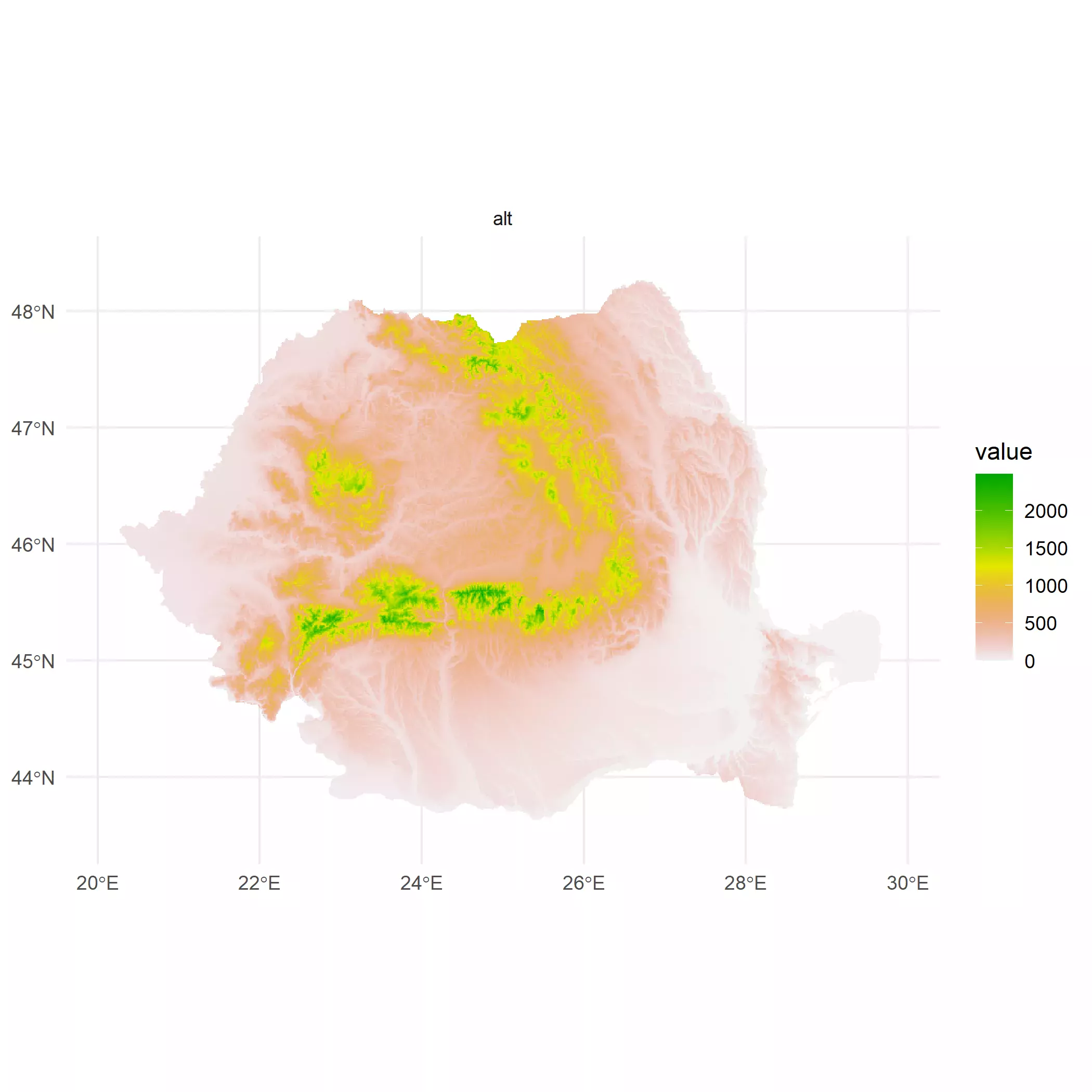Next step is to calculate the hillshade. Royé has a very detailed discussion here, so I would not go into details. Basically what we want to create is a layer that approximates the potential “texture” of the surface based on the elevation and the sun position. This is straightforward with `terra::terrain()` and `terra::shade()` functions:

``````

slope <- terrain(r, "slope", unit = "radians")
aspect <- terrain(r, "aspect", unit = "radians")
hill <- shade(slope, aspect, 30, 270)

# normalize names

# Hillshading, but we need a palette
pal_greys <- hcl.colors(1000, "Grays")

ggplot() +
geom_spatraster(data = hill) +
scale_fill_gradientn(colors = pal_greys, na.value = NA)
#> SpatRaster resampled to ncells = 501501
``````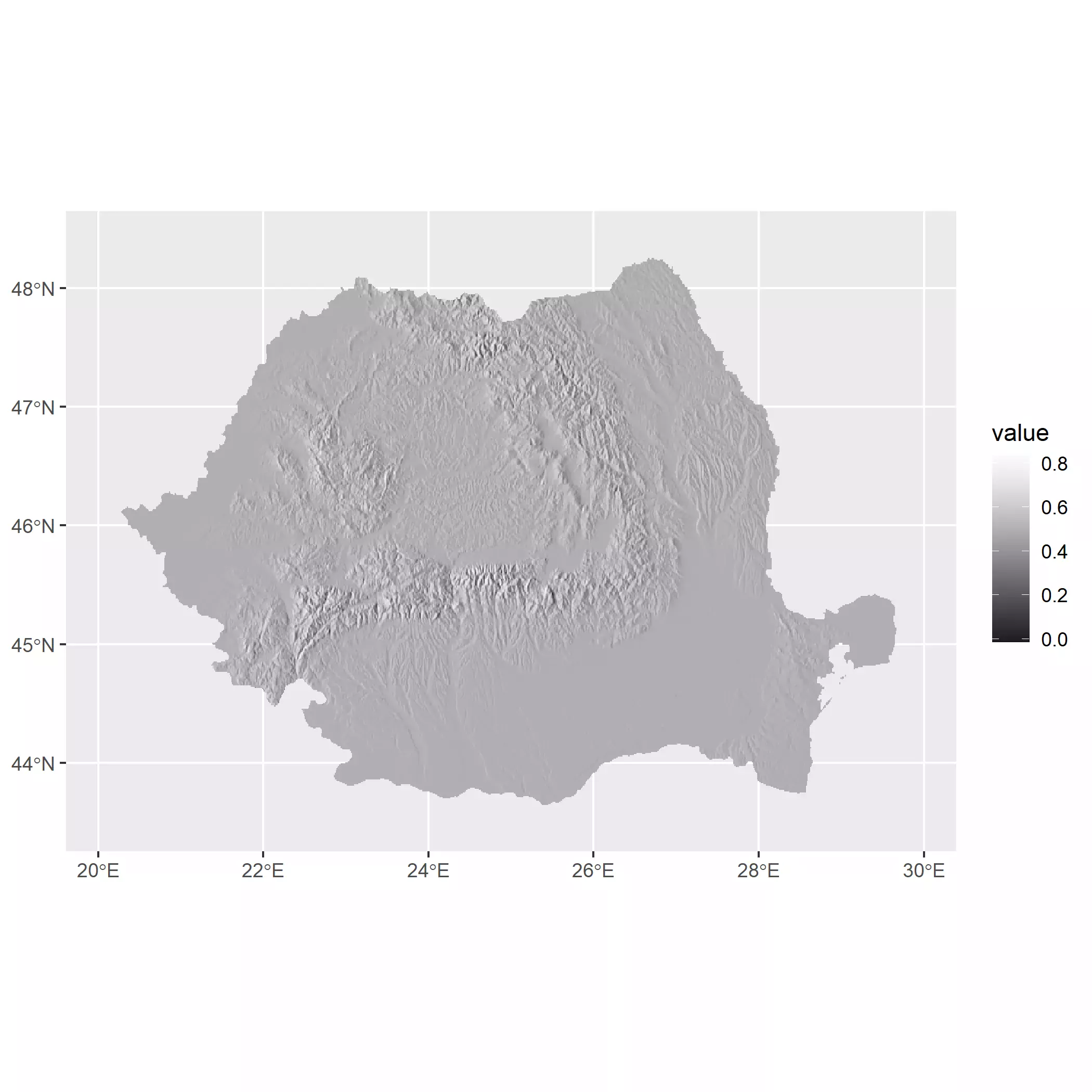We can also do the following hack to avoid the use of a `scale_fill_*` (via `ggplot2` or via `ggnewscale::new_scale_fill()`):

• Select a vector of colors (in this post `pal_greys`).
• Extract the values of the raster and reescale them to the length of the palette (`c(1, 1000)`).
• Round those rescaled values to the nearest integer. So we would have a index indicating which value of `pal_greys` should be mapped to each cell.
• Now use the parameter `fill` on the `geom_` instead of using the scale.

An additional note is that `geom_spatraster()` has a parameter `maxcell` that would perform a spatial resampling if the raster has more cells than `maxcell`. This is for optimization (note that `terra::plot()` has the same setup and that the users often forgot about it), but we can force to plot all the cells by using `maxcell = Inf`. On this approach for using `fill` the value `maxcell` needs to be effectively set to `Inf` to ensure that the number of color values and the number of cells is the same.

``````
# Use a vector of colors

index <- hill %>%
mutate(index_col = rescale(shades, to = c(1, length(pal_greys)))) %>%
mutate(index_col = round(index_col)) %>%
pull(index_col)

# Get cols
vector_cols <- pal_greys[index]

# Need to avoid resampling
# and dont use aes

hill_plot <- ggplot() +
geom_spatraster(
data = hill, fill = vector_cols, maxcell = Inf,
alpha = 1
)

hill_plot
``````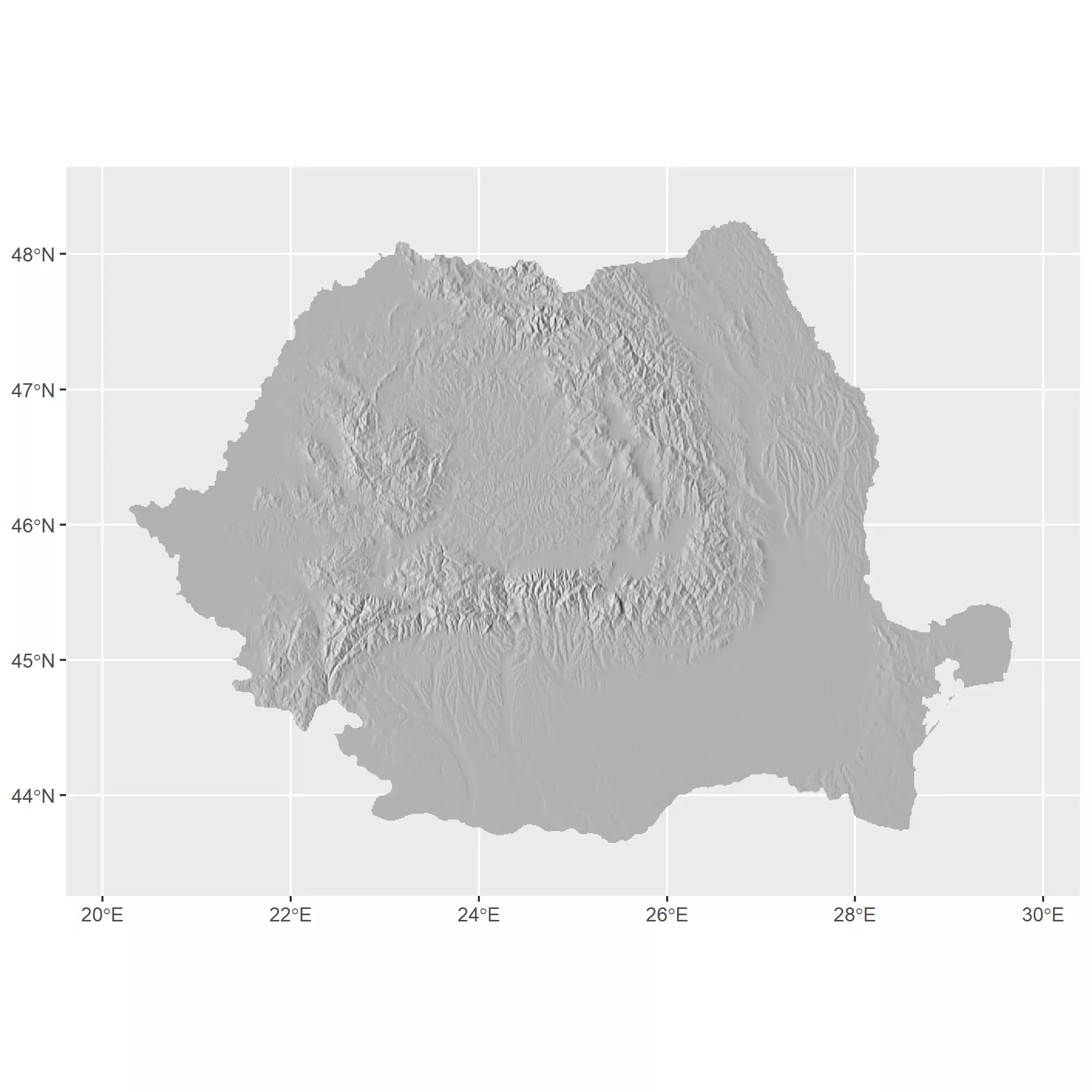## Selecting colors

The selection of colors for elevation maps is a key aspect when designing this kind of visualization since colors can be confused with environmental phenomena (Patterson and Jenny, 2011). For example, by convention green colors are associated to low elevations while orange, browns and whites are associared to high elevations on some of the most common elevation palettes (aka hypsometric tints). See for example the Wikipedia Topographic maps conventions.

This is not ideal, since greens can be confused with forests, for example, so an elevation map of desertic areas would not be appropiated with a green-brown-white color scheme.

There is an additional point to take into account when designing color palettes for maps. A regular gradient would just interpolate colors assuming that the distance among colors is the same:

``````

autoplot(r) +
``````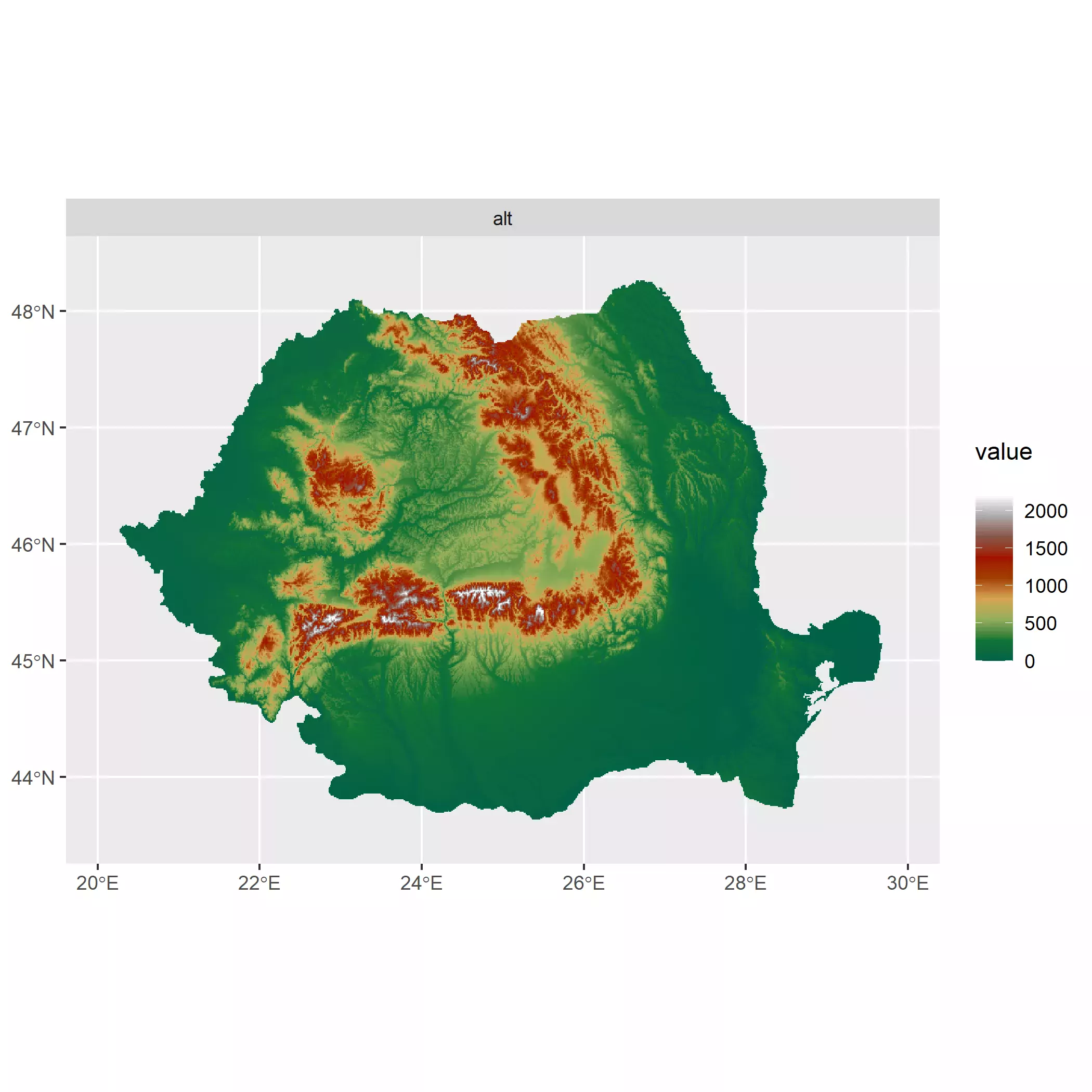For that reason, tidyterra provides additional gradients whose colors are placed unevenly with the goal of providing a better understanding of the maps:

``````

autoplot(r) +
``````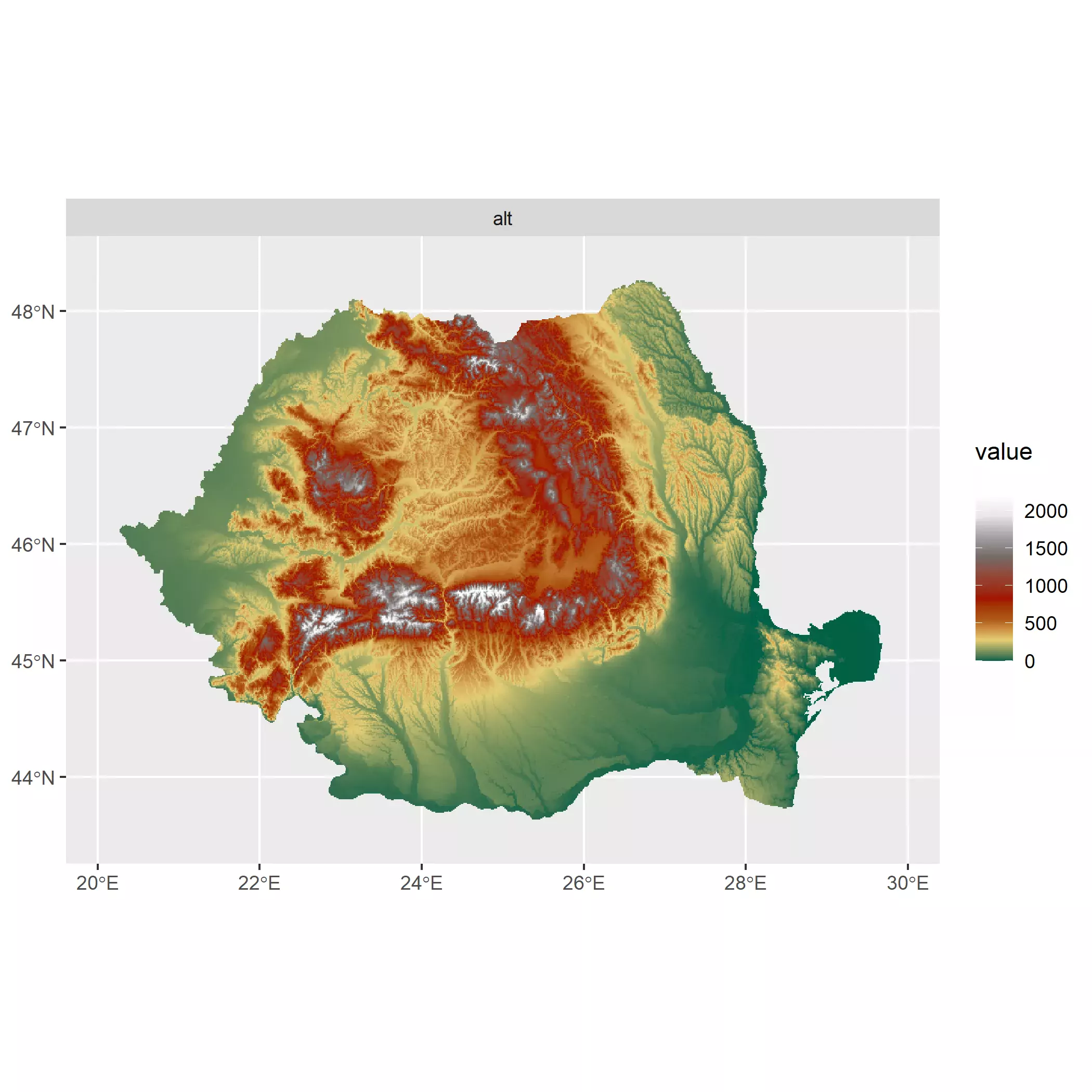Can you notice the difference? In the first map greens are the dominant color. However greens are representing a wide range of elevations (0-750 meters) that correspond with most of the territory. In terms of perception, we won’t be clearly spotting elevation differences in the center of the country, while with the uneven gradient greens only correspond to the range (0 - 250 meters) and the overall perception of elevation improves. Note that the only difference between plots is exclusively the color palette.

For producing our map we are going to assess visually the result of a selection of palettes provided by `tidyterra`. We use here the version `tidyterra::scale_fill_hypso_tint_c()` instead of `tidyterra::scale_fill_hypso_c()` for taking advantage of the uneven color gradients.

A downside of using this scales is that we need also to adjust the `limits` argument of the functions to make `ggplot2` aware of the limits of the value of the raster. This is easily achieved with `terra::minmax()` but I added an extra touch rounding up and down the range of values to the nearest 500.

``````
# Try some options, but we need to be aware of the values of our raster

r_limits <- minmax(r) %>% as.vector()

# Rounded to lower and upper 500
r_limits <- c(floor(r_limits / 500), ceiling(r_limits / 500)) * 500

# And making min value to 0.
r_limits <- pmax(r_limits, 0)

# Compare
minmax(r) %>% as.vector()
#>     0 2481
r_limits
#>     0 2500

# Now lets have some fun with scales from tidyterra

elevt_test <- ggplot() +
geom_spatraster(data = r)

# Create a helper function

plot_pal_test <- function(pal) {
elevt_test +
scale_fill_hypso_tint_c(
limits = r_limits,
palette = pal
) +
ggtitle(pal) +
theme_minimal()
}

plot_pal_test("etopo1_hypso")
plot_pal_test("dem_poster")
plot_pal_test("spain")
plot_pal_test("pakistan")
plot_pal_test("utah_1")
plot_pal_test("wiki-2.0_hypso")
``````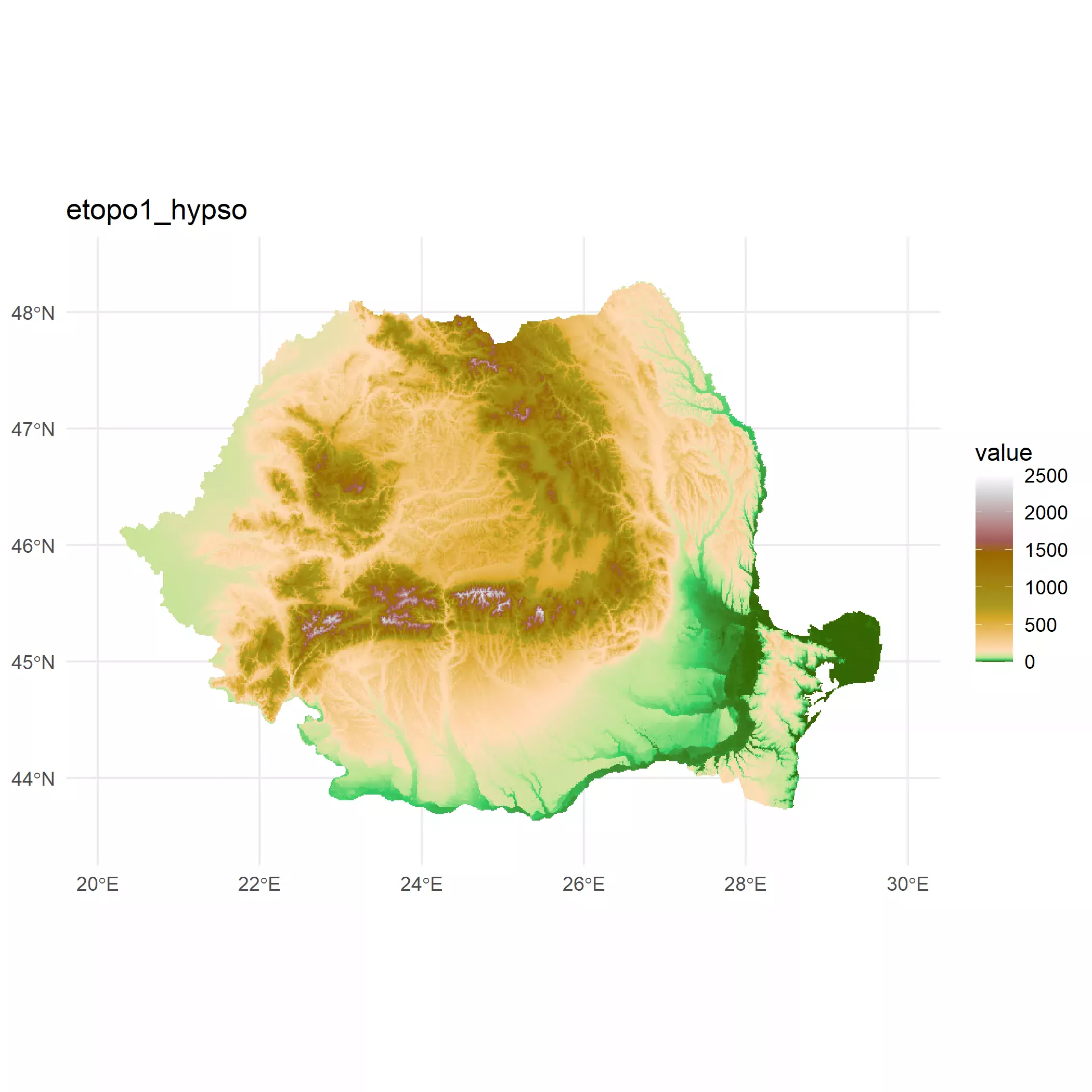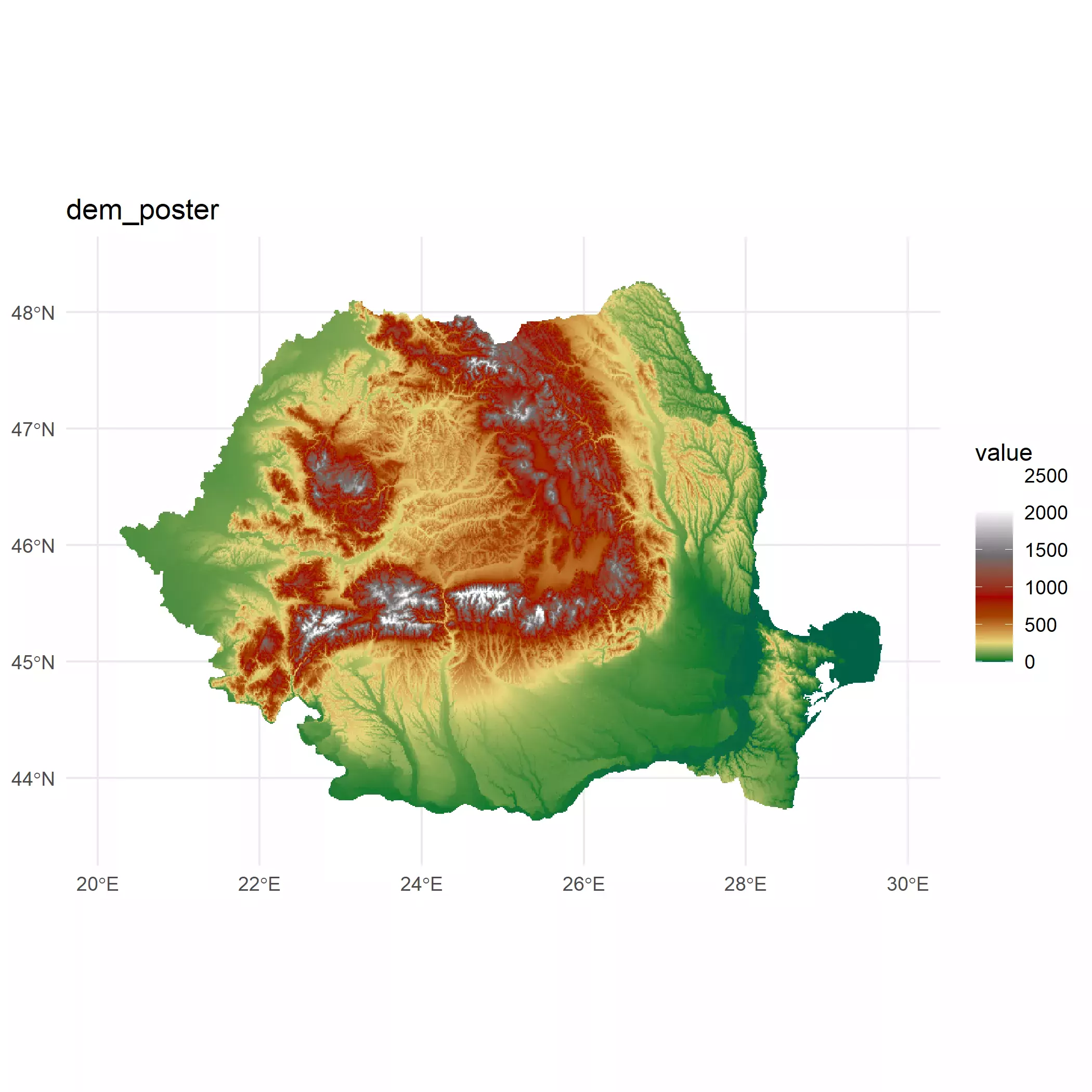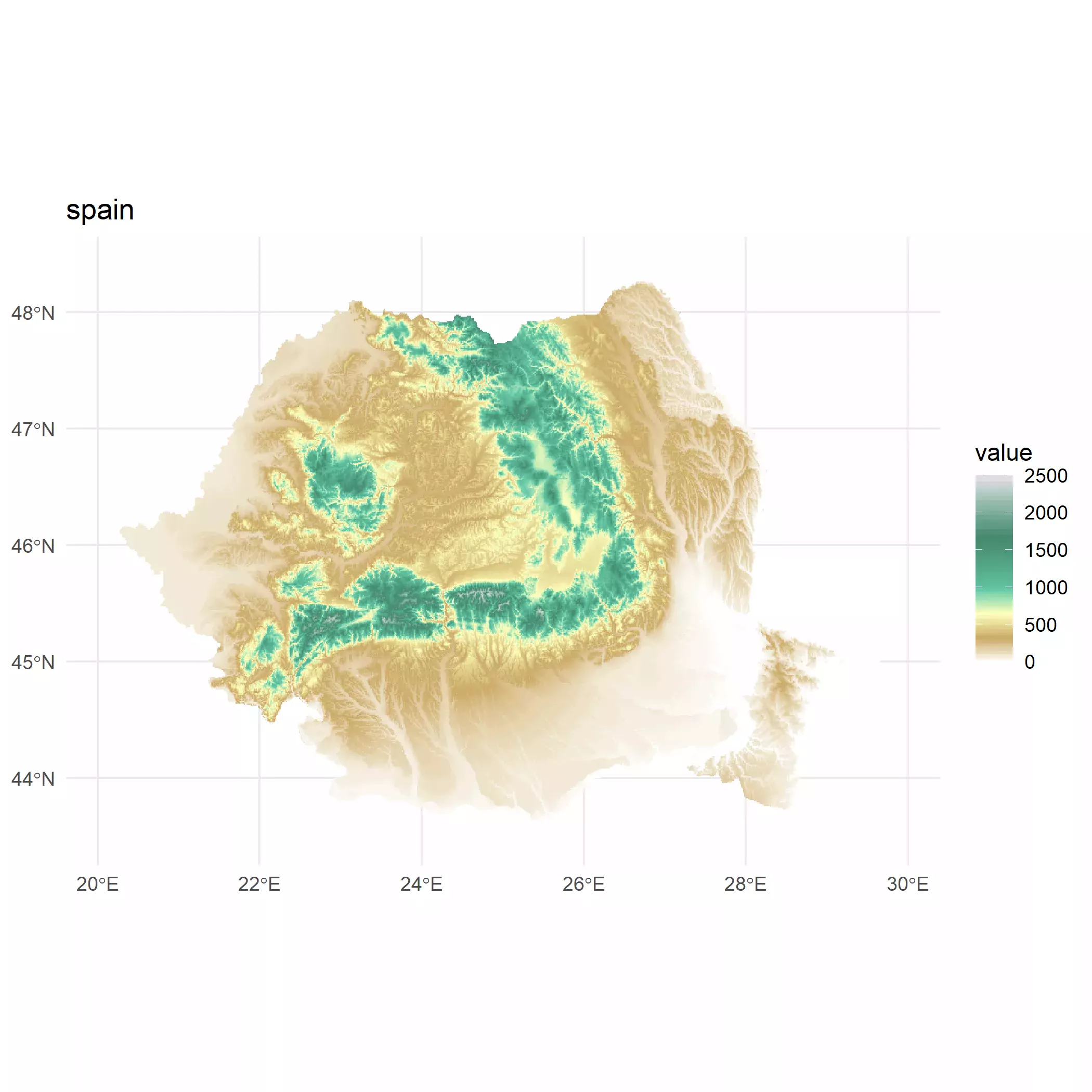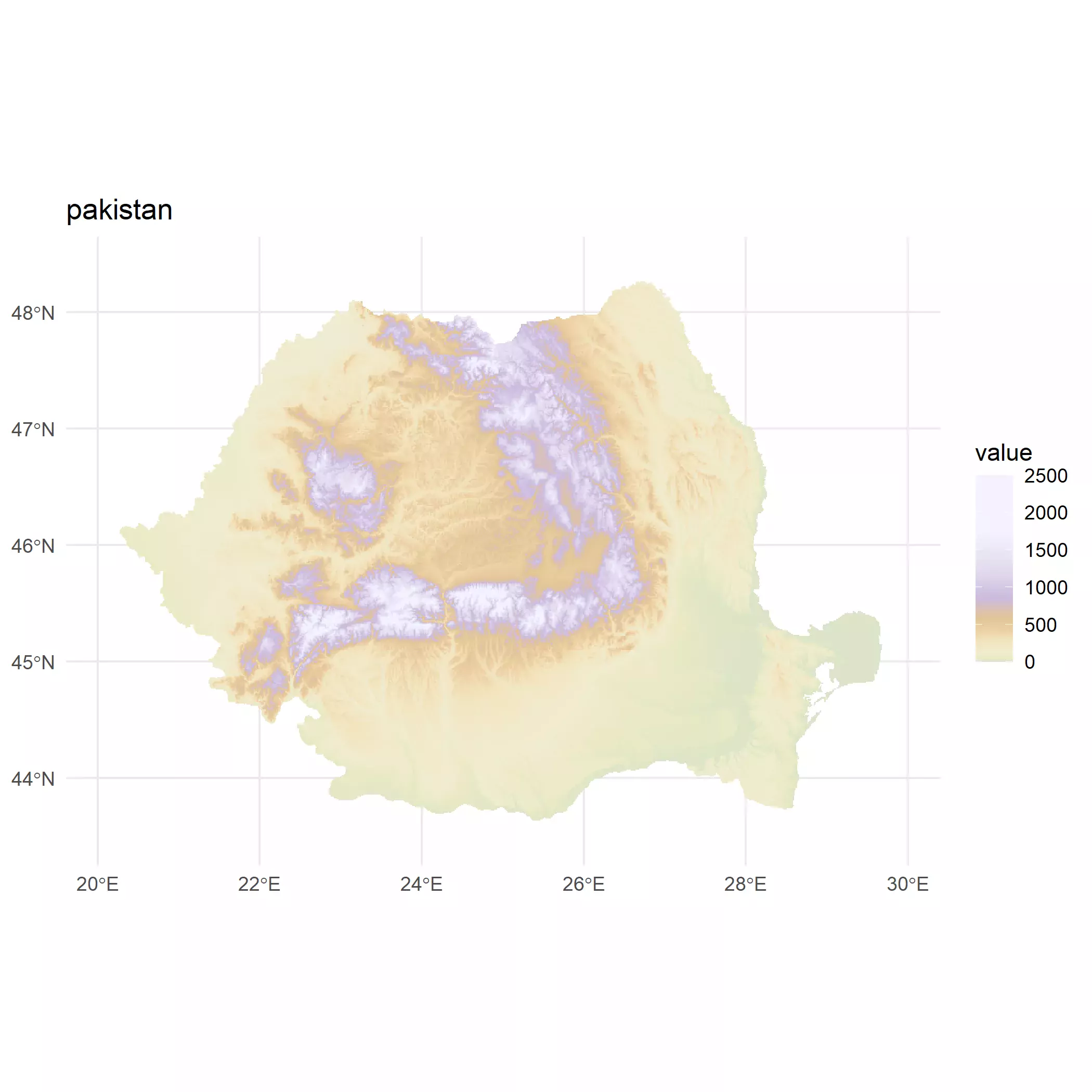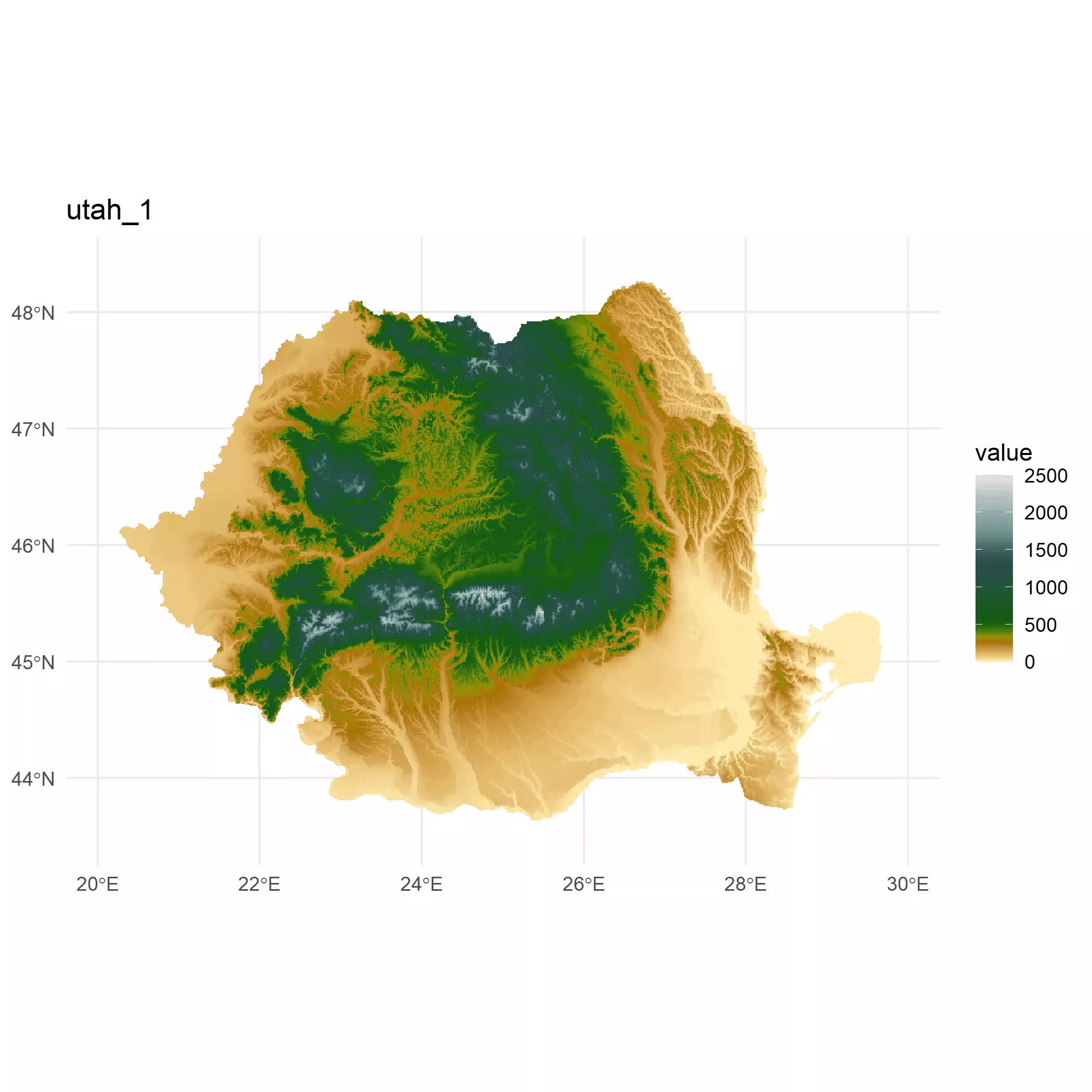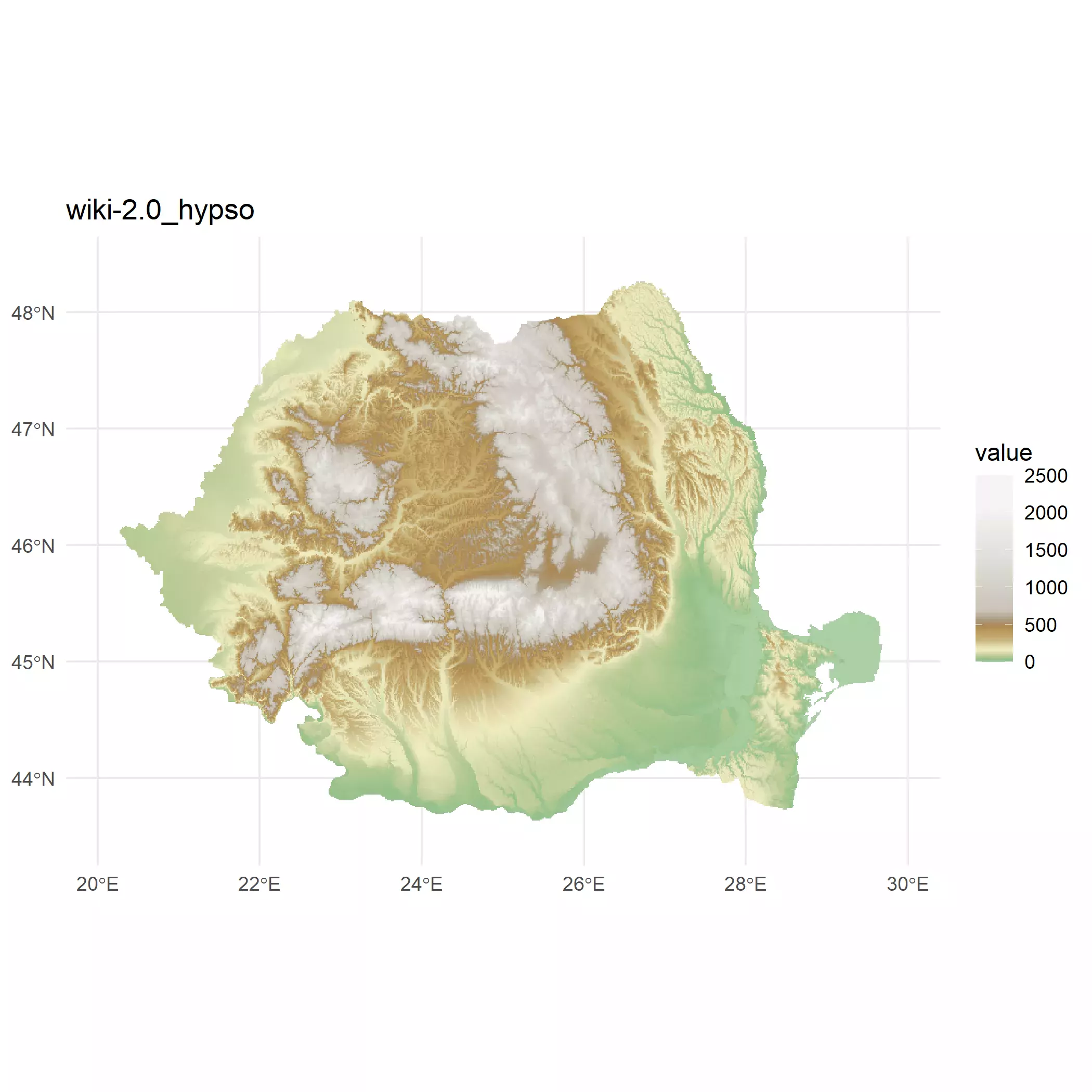I finally selected for my plot the `"dem_poster"` palette, but this is completely a personal choice. You should select the palette you feel more comfortable with. See the full range of color palettes provided by `tidyterra` here.

## Final plot

So now it is time to blend both the hillshade layer and the altitude layer using some level of `alpha` on the upper layer.

``````
base_plot <- hill_plot +
# Avoid resampling with maxcell
geom_spatraster(data = r, maxcell = Inf) +
scale_fill_hypso_tint_c(
limits = r_limits,
palette = "dem_poster",
alpha = 0.4,
labels = label_comma(),
# For the legend I use custom breaks
breaks = c(
seq(0, 500, 100),
seq(750, 1500, 250),
2000
)
)

base_plot
``````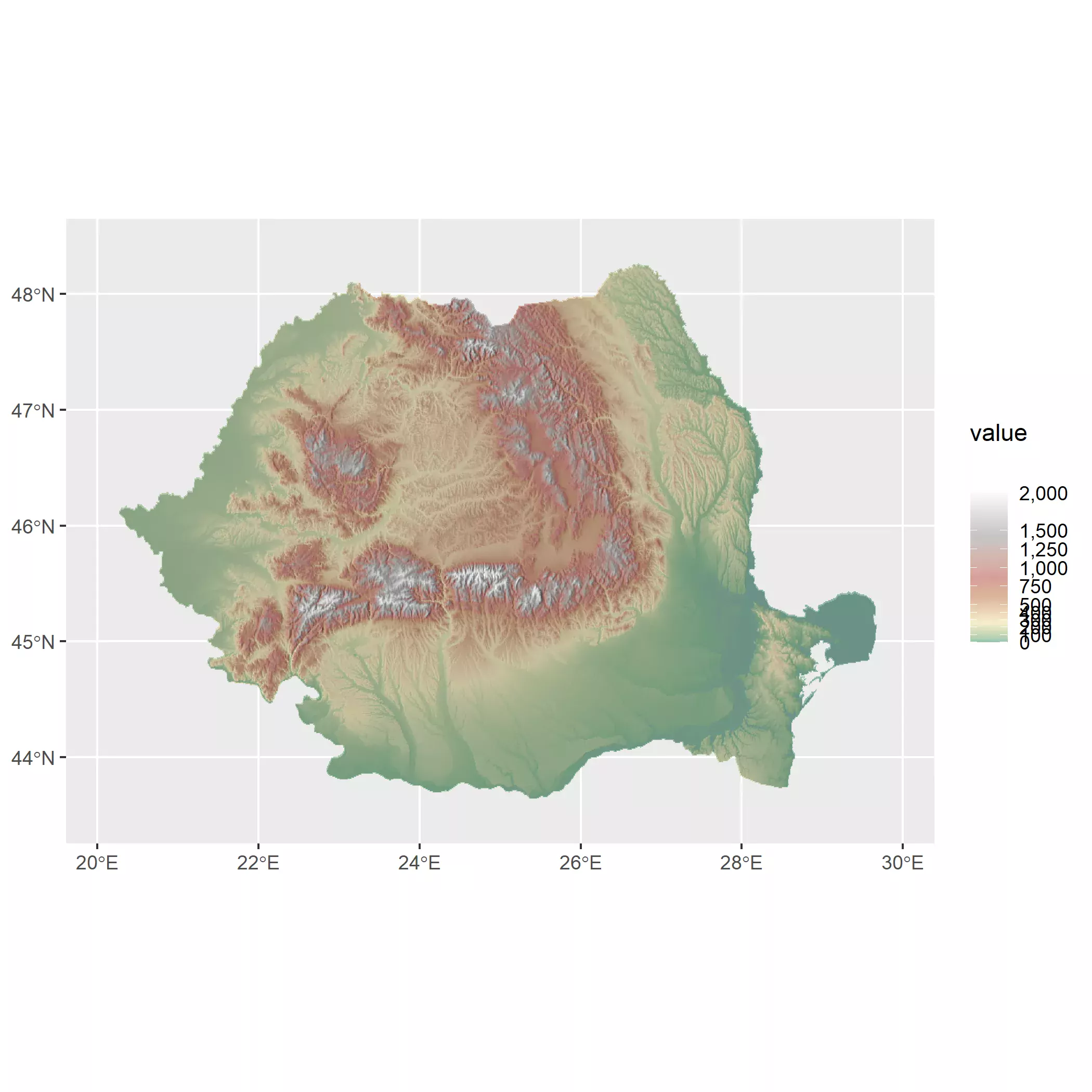And with a bit of trickery and theming we can have our final map. First we load a font from Google with a custom function:

``````myload_fonts <- function(fontname, family,
fontdir = tempdir()) {
fontname_url <- utils::URLencode(fontname)
fontzip <- tempfile(fileext = ".zip")
fontzip,
quiet = TRUE,
mode = "wb"
)
unzip(fontzip,
exdir = fontdir,
junkpaths = TRUE
)

paths <- list(
regular = "Regular.ttf",
bold = "Bold.ttf",
italic = "Italic.ttf",
bolditalic = "BoldItalic.ttf"
)

namefile <- gsub(" ", "", fontname)
paths_end <- file.path(
fontdir,
paste(namefile, paths, sep = "-")
)

names(paths_end) <- names(paths)

regular = paths_end["regular"],
bold = paths_end["bold"],
italic = paths_end["italic"],
bolditalic = paths_end["bolditalic"]
)

return(invisible())
}
``````

And now we theme it:

``````# Theming
showtext::showtext_auto()

base_text_size <- 30

base_plot +
# Change guide
guides(fill = guide_legend(
title = "   m.",
direction = "horizontal",
nrow = 1,
keywidth = 1.75,
keyheight = 0.5,
label.position = "bottom",
title.position = "right",
override.aes = list(alpha = 1)
)) +
labs(
title = "Elevation of Romania",
subtitle = "Hillshade and hypsometric tint blend",
caption = paste0(
"@dhernangomez using tidyterra, ggplot2, geodata R packages.",
" Data: Shuttle Radar Topography Mission (SRTM)"
)
) +
theme_minimal(base_family = "notoserif") +
theme(
plot.background = element_rect("grey97", colour = NA),
plot.margin = margin(20, 20, 20, 20),
plot.caption = element_text(size = base_text_size * 0.5),
plot.title = element_text(face = "bold", size = base_text_size * 1.4),
plot.subtitle = element_text(
margin = margin(b = 10),
size = base_text_size
),
axis.text = element_text(size = base_text_size * 0.7),
legend.position = "bottom",
legend.title = element_text(size = base_text_size * 0.8),
legend.text = element_text(size = base_text_size * 0.8),
legend.key = element_rect("grey50"),
legend.spacing.x = unit(0, "pt")
)
``````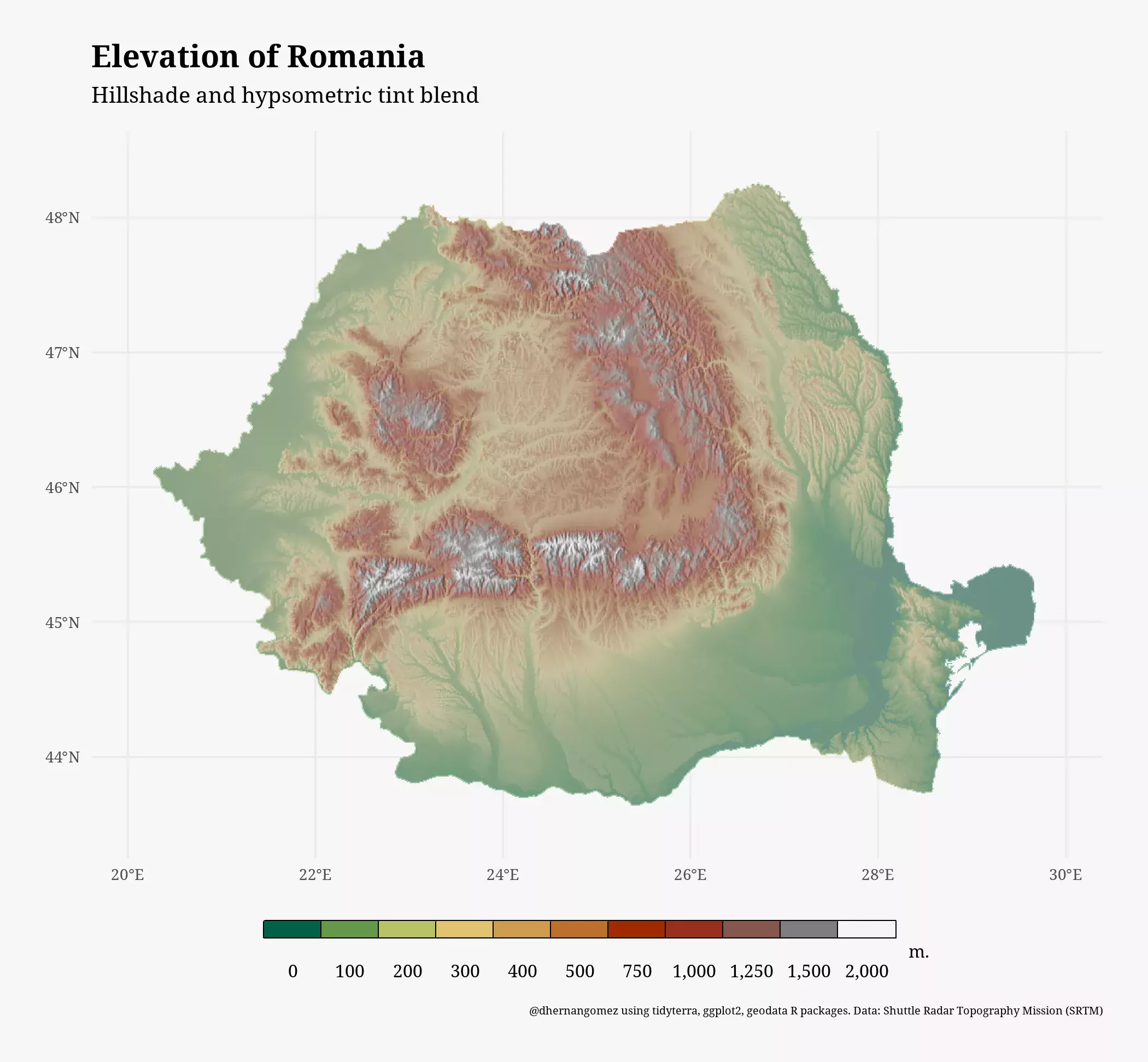Patterson T, Jenny B (2011). “The Development and Rationale of Cross-blended Hypsometric Tints.” Cartographic Perspectives, 31–46. https://doi.org/10.14714/CP69.20

Grossenbacher T (2016). “Beautiful thematic maps with ggplot2 (only).” https://timogrossenbacher.ch/2016/12/beautiful-thematic-maps-with-ggplot2-only/.